#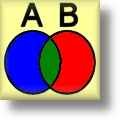Venn Diagram Worksheets

## Dynamically Created Venn Diagram Worksheets

These Venn Diagram Worksheets are great for testing students on set theory and working with Venn Diagrams. These Venn Diagram Worksheets are dynamically created for you so you will have an endless supply of quality material to use in the classroom.

The "Venn Diagram Rules Handout Worksheet" is great for reinforcing the rules of set theory.

The "Venn Diagram Templates for Two and Three Set" are great handouts for the students to use.

A detailed description of each Venn Diagram Worksheet is provided below.

Click here for a Detailed Description of all the Venn Diagram Worksheets.

## Quick Link for All Venn Diagram Worksheets

Click the image to be taken to that Venn Diagram Worksheet.

##### Venn Diagram WorksheetsVenn Diagram Template Using Two Sets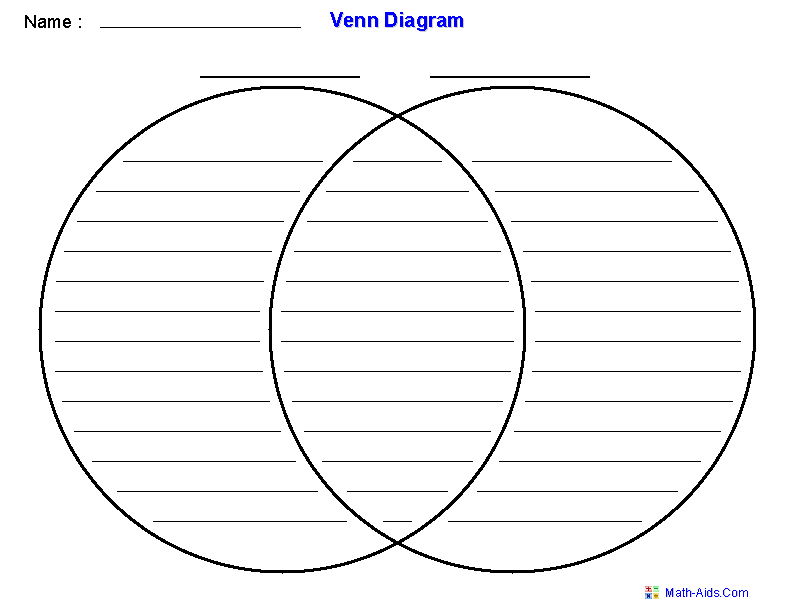##### Venn Diagram WorksheetsVenn Diagram Template Using Three Sets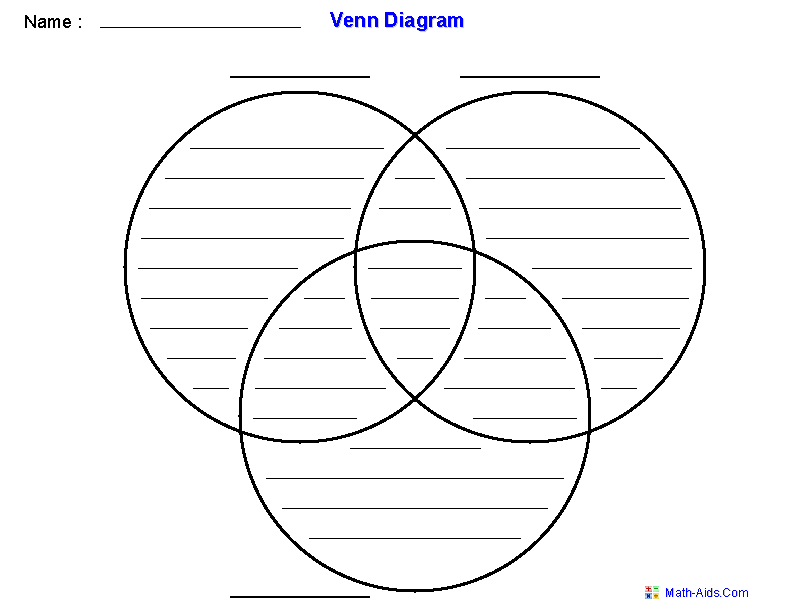##### Venn Diagram WorksheetsSet Theory DefinitionsHandout Worksheet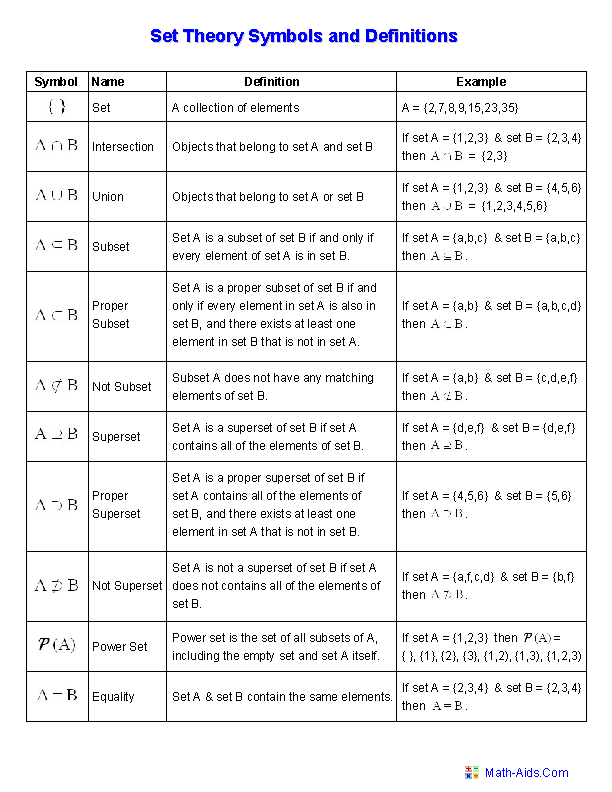##### Venn Diagram WorksheetsShade the RegionsUsing Two Sets##### Venn Diagram WorksheetsShade the RegionsUsing Three Sets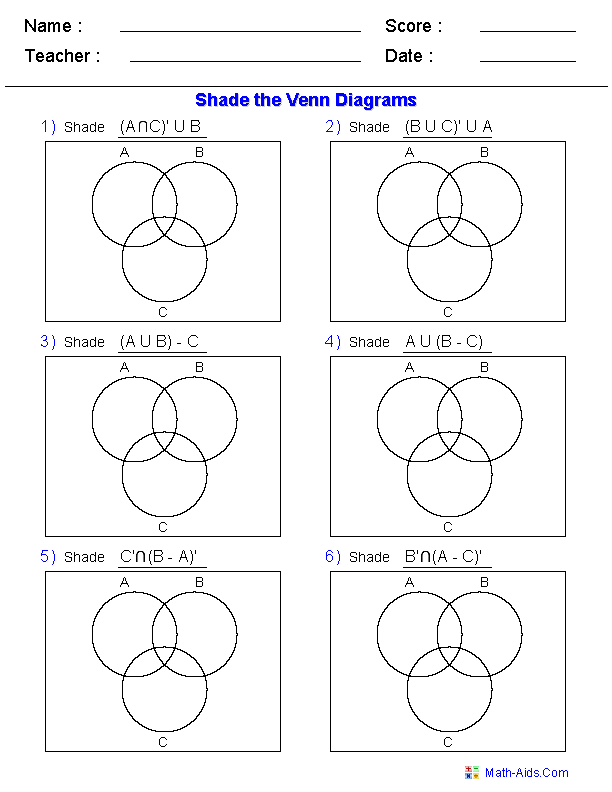##### Venn Diagram WorksheetsName the Shaded RegionsUsing Two Sets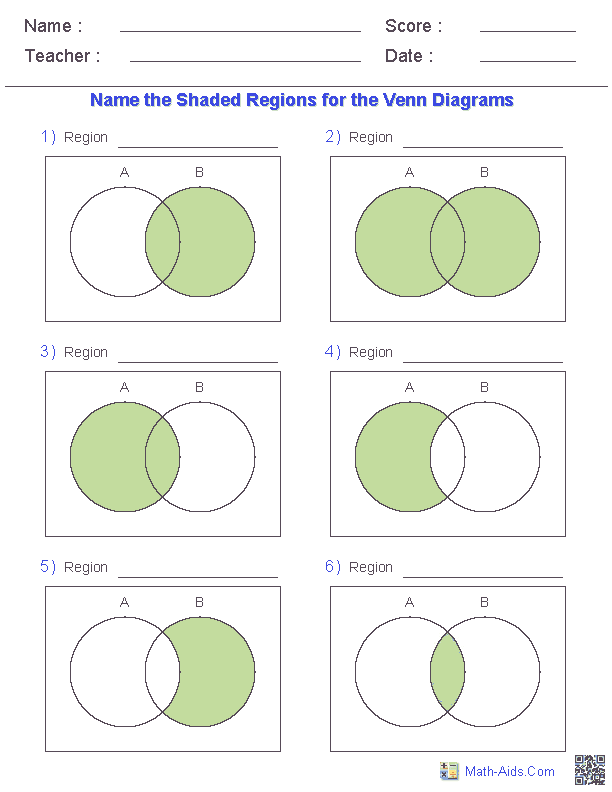##### Venn Diagram WorksheetsName the Shaded RegionsUsing Three Sets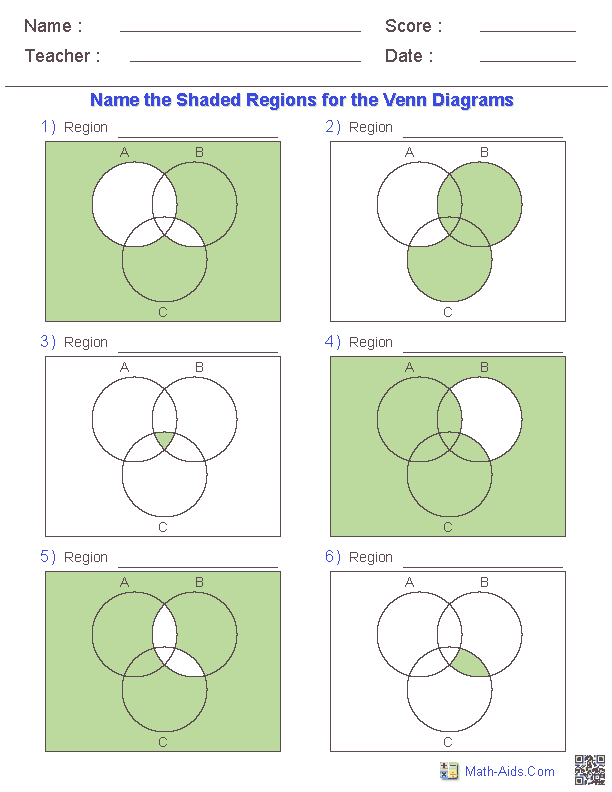##### Venn Diagram WorksheetsSet Notation ProblemsUsing Two Sets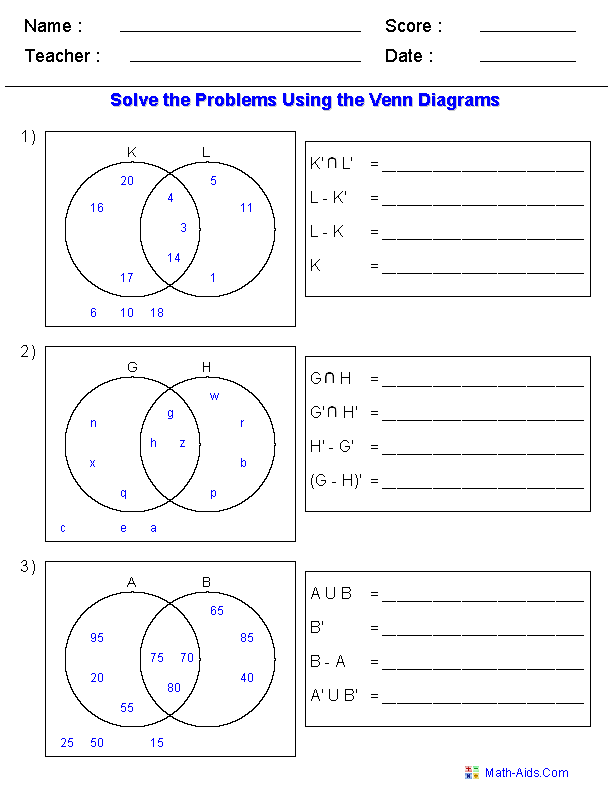##### Venn Diagram WorksheetsSet Notation ProblemsUsing Three Sets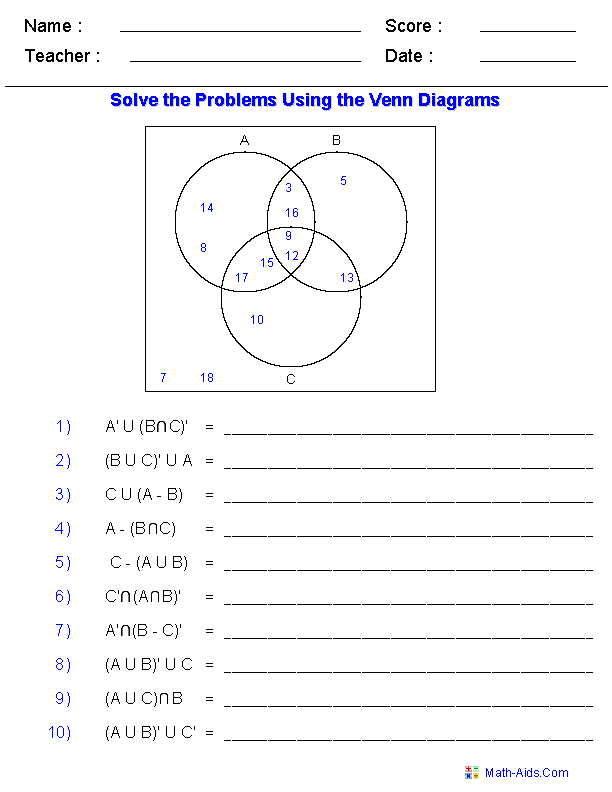##### Venn Diagram WorksheetsWord ProblemsUsing Two Sets##### Venn Diagram WorksheetsWord ProblemsUsing Three Sets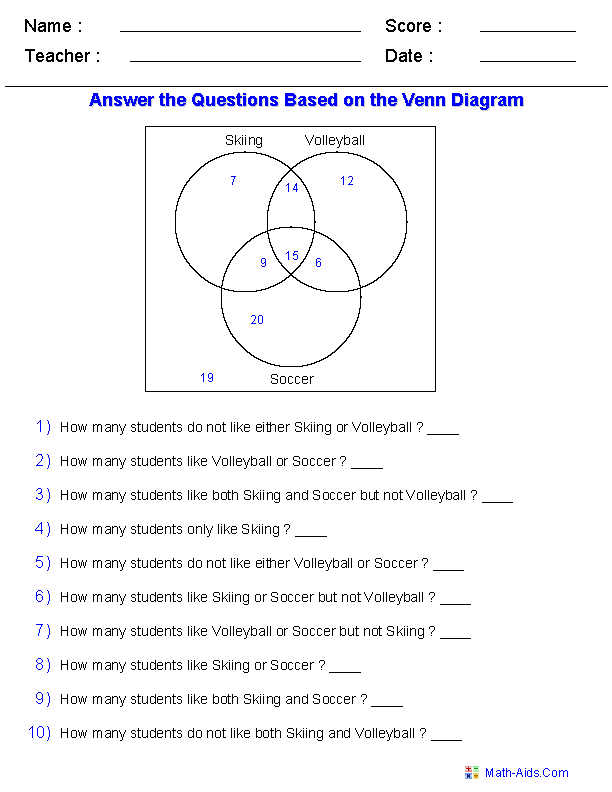Recommended Videos

## Detailed Description for All Venn Diagram Worksheets

Venn Diagram Template Using Two Sets
These Venn Diagram Worksheets are great templates using two sets. Use them for practicing Venn Diagrams to solve different sets, unions, intersections, and complements.

Venn Diagram Template Using Three Sets
These Venn Diagram Worksheets are great templates using three sets. Use them for practicing Venn Diagrams to solve different sets, unions, intersections, and complements.

Set Theory Definitions Handout
These Venn Diagram Worksheets are great handouts for reinforcing the symbols and definitions used in Set Theory.

Shade the Regions Using Two Sets Worksheet
These Venn Diagram Worksheets are great for practicing shading the regions of different sets, unions, intersections, and complements using two sets. These Venn Diagram Worksheets will produce 6 Venn Diagrams for the students to shade.

Shade the Regions Using Three Sets Worksheet
These Venn Diagram Worksheets are great for practicing identifying the shaded regions of different sets, unions, intersections, and complements of three sets. These Venn Diagram Worksheets use advanced combinations of unions, intersections, relative complements and complements of sets. You may select to use standard sets, complements of sets or both. These Venn Diagram Worksheets will produce 6 Venn Diagrams for the students to shade.

Name the Shaded Regions Using Two Sets Worksheet
These Venn Diagram Worksheets are great for practicing identifying the shaded regions of different sets, unions, intersections, and complements of two sets. These Venn Diagram Worksheets use advanced combinations of unions, intersections, relative complements and complements of sets. You may select to use standard sets, complements of sets or both. These Venn Diagram Worksheets will produce 6 shaded Venn Diagrams for the students to name.

Name the Shaded Regions Using Three Sets Worksheet
These Venn Diagram Worksheets are great for practicing identifying the shaded regions of different sets, unions, intersections, and complements of three sets. These Venn Diagram Worksheets use advanced combinations of unions, intersections, relative complements and complements of sets. You may select to use standard sets, complements of sets or both. These Venn Diagram Worksheets will produce 6 shaded Venn Diagrams for the students to name.

Set Notation Problems Using Two Sets Worksheets
These Venn Diagram Worksheets are great for practicing solving set notation problems of different sets, unions, intersections, and complements with two sets. You may select to use single sets, unions, intersection, and relative complements of sets. These Venn Diagram Worksheets will produce three problems with a maximum of 4 questions for each Venn Diagram for the students to answer.

Set Notation Problems Using Three Sets Worksheets
These Venn Diagram Worksheets are great for practicing solving set notation problems of different sets, unions, intersections, and complements with three sets. These Venn Diagram Worksheets use advanced combinations of unions, intersections, relative complements and complements of sets. You may select to use standard sets, complements of sets or both. These Venn Diagram Worksheets will produce 10 questions on a single Venn Diagram for the students to answer.

Word Problems Using Two Sets Worksheet
These Venn Diagram Worksheets are great for working word problems of different sets, unions, intersections, and complements using two sets. These Venn Diagram Worksheets will produce 10 questions on a single Venn Diagram for the students to answer.

Word Problems Using Three Sets Worksheet
These Venn Diagram Worksheets are great for working word problems of different sets, unions, intersections, and complements using three sets. These Venn Diagram Worksheets will produce 10 questions on a single Venn Diagram for the students to answer.